# SVGBasics

Turn SVG On

## Adding Gloss - Using Filters for Lighting

### Lighting and Light sources

Lighting is done in SVG with the 'feDiffuseLighting' and 'feSpecularLighting' filter effects. These follow established models for the way lighting is calculated in computer graphics. 'feSpecularLighting'
Earlier I mentioned that feComposite should be used to add the specular lighting to the original image.
Specifically, to add the two images, use feComposite with the arithmetic operator and k1=0, k2=1, k3=1, k4=0.
The tag looks like this <feComposite in="SourceGraphic" in2="specOut" operator="arithmetic" k1="0" k2="1" k3="1" k4="0"/>. Just replace 'specOut' with the name of the output from your specular lighting stage. If that's all you need, you can just copy and paste the tag and skip the following more detailed explanation.
The output from the arithmetic operator is computed as

```result = k1*in1*in2 + k2*in1 + k3*in2 + k4
```

where k1 .. k4 are attributes of feComposite (which are only used for this purpose), in1 and in2 are pixel colour values from each of the in and in2 input images, and the operators '*' and '+' happen for each of the RGB and Alpha channels independently.
Obviously, setting k2 and k3 to 1 with k1 and k4 at 0 makes the equation simplify to
```result = in1 + in2
```

This performs the addition of the two images. Since the output of feSpecularLighting is meant to be added to the original image, we specify 'SourceGraphic' as one input ('in') to get the original image and the result of the feSpecularLighting effect for the other input ('in2'). If you applied feSpecularLighting to some other intermediate image, you'd specify that image's id as in and the result of feSpecularLighting as in2.
Here are examples of specular lighting with lights generally originating from the bottom right and generally pointing toward the top left area. The parameters and effects of each light source are different, so they aren't all equivalent.

### fePointLight Example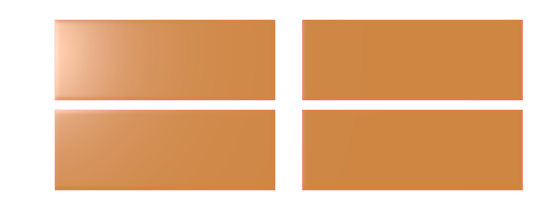<?xml version="1.0" standalone="no"?>
<!DOCTYPE svg PUBLIC "-//W3C//DTD SVG 1.1//EN" "http://www.w3.org/Graphics/SVG/1.1/DTD/svg11.dtd">

<svg viewBox = "0 0 1100 400" version = "1.1">
<desc>
Filter example
</desc>
<filter id = "i1">
<feGaussianBlur in = "SourceAlpha" stdDeviation = "4" result = "blur1"/>
<feSpecularLighting result = "specOut" in = "blur1" specularExponent = "20" lighting-color = "#bbbbbb">
<fePointLight x = "50" y = "100" z = "200"/>
</feSpecularLighting>
<feComposite in = "SourceGraphic" in2 = "specOut" operator = "arithmetic" k1 = "0" k2 = "1" k3 = "1" k4 = "0"/>
</filter>
<g stroke = "tomato" fill = "peru" filter = "url(#i1)">
<rect x = "10%" y = "10%" width = "40%" height = "40%"/>
<rect x = "55%" y = "10%" width = "40%" height = "40%"/>
<rect x = "10%" y = "55%" width = "40%" height = "40%"/>
<rect x = "55%" y = "55%" width = "40%" height = "40%"/>
</g>
</svg>

### feDistantLight Example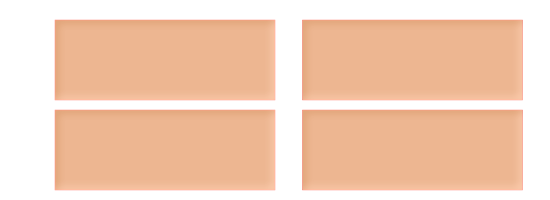<?xml version="1.0" standalone="no"?>
<!DOCTYPE svg PUBLIC "-//W3C//DTD SVG 1.1//EN" "http://www.w3.org/Graphics/SVG/1.1/DTD/svg11.dtd">

<svg viewBox = "0 0 1100 400" version = "1.1">
<desc>
Filter example
</desc>
<filter id = "i1">
<feGaussianBlur in = "SourceAlpha" stdDeviation = "8" result = "blur1"/>
<feSpecularLighting result = "specOut" in = "blur1" specularConstant = "1.2" specularExponent = "12" lighting-color = "#bbbbbb">
<feDistantLight azimuth = "45" elevation = "45"/>
</feSpecularLighting>
<feComposite in = "SourceGraphic" in2 = "specOut" operator = "arithmetic" k1 = "0" k2 = "1" k3 = "1" k4 = "0"/>
</filter>
<g stroke = "tomato" fill = "peru" filter = "url(#i1)">
<rect x = "10%" y = "10%" width = "40%" height = "40%"/>
<rect x = "55%" y = "10%" width = "40%" height = "40%"/>
<rect x = "10%" y = "55%" width = "40%" height = "40%"/>
<rect x = "55%" y = "55%" width = "40%" height = "40%"/>
</g>
</svg>

### feSpotLight Example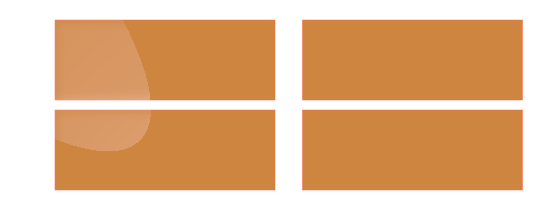<?xml version="1.0" standalone="no"?>
<!DOCTYPE svg PUBLIC "-//W3C//DTD SVG 1.1//EN" "http://www.w3.org/Graphics/SVG/1.1/DTD/svg11.dtd">

<svg viewBox = "0 0 1100 400" version = "1.1">
<filter id = "i1">
<feGaussianBlur in = "SourceAlpha" stdDeviation = "8" result = "blur1"/>
<feSpecularLighting result = "specOut" in = "blur1" specularConstant = "1.2" specularExponent = "12" lighting-color = "#bbbbbb">
<feSpotLight x = "800" y = "800" z = "400" pointsAtX = "0" pointsAtY = "0" pointsAtZ = "0" limitingConeAngle = "9"/>
</feSpecularLighting>
<feComposite in = "SourceGraphic" in2 = "specOut" operator = "arithmetic" k1 = "0" k2 = "1" k3 = "1" k4 = "0"/>
</filter>
<g stroke = "tomato" fill = "peru" filter = "url(#i1)">
<rect x = "10%" y = "10%" width = "40%" height = "40%"/>
<rect x = "55%" y = "10%" width = "40%" height = "40%"/>
<rect x = "10%" y = "55%" width = "40%" height = "40%"/>
<rect x = "55%" y = "55%" width = "40%" height = "40%"/>
</g>
</svg>

### 'feDiffuseLighting'

Diffuse lighting uses the Phong lighting model.
The feDiffuseLighting effect produces an image with Alpha=1.0 everywhere. It is meant to be combined with the original image by using the arithmetic operator of the feCompisite to multiply the diffuse lighting with the original image. This is done with a tag like this <feComposite in="SourceGraphic" in2="specOut" operator="arithmetic" k1="1" k2="0" k3="0" k4="0"/>.
The resulting image pixels are calculated as

```result = k1*in1*in2 + k2*in1 + k3*in2 + k4
```

where k1 .. k4 are attributes of feComposite (which are only used for this purpose), in1 and in2 are pixel colour values from each of the in and in2 input images, and the operators '*' and '+' happen for each of the RGB and Alpha channels independently.
For our diffuse lighting tag, k1=1 and the rest are 0, so the equation becomes
```result = in1*in2
```

Here are examples analogous to those given for specular lighting. Notice that diffuse lighting generally has a more subdued effect.

### fePointLight Example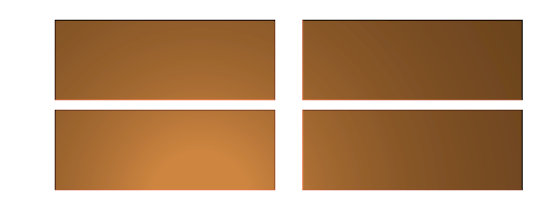<?xml version="1.0" standalone="no"?>
<!DOCTYPE svg PUBLIC "-//W3C//DTD SVG 1.1//EN" "http://www.w3.org/Graphics/SVG/1.1/DTD/svg11.dtd">

<svg viewBox = "0 0 1100 400" version = "1.1">
<desc>
Filter example
</desc>
<filter id = "i1">
<feDiffuseLighting result = "diffOut" in = "SourceGraphic" diffuseConstant = "1.2" lighting-color = "white">
<fePointLight x = "400" y = "400" z = "150" pointsAtX = "0" pointsAtY = "0" pointsAtZ = "0"/>
</feDiffuseLighting>
<feComposite in = "SourceGraphic" in2 = "diffOut" operator = "arithmetic" k1 = "1" k2 = "0" k3 = "0" k4 = "0"/>
</filter>
<g stroke = "tomato" fill = "peru" filter = "url(#i1)">
<rect x = "10%" y = "10%" width = "40%" height = "40%"/>
<rect x = "55%" y = "10%" width = "40%" height = "40%"/>
<rect x = "10%" y = "55%" width = "40%" height = "40%"/>
<rect x = "55%" y = "55%" width = "40%" height = "40%"/>
</g>
</svg>

### feDistantLight Example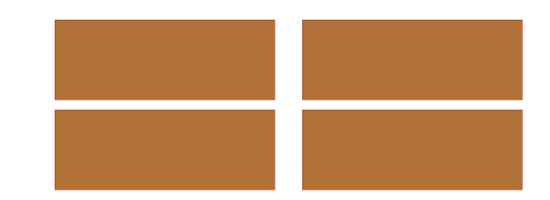<?xml version="1.0" standalone="no"?>
<!DOCTYPE svg PUBLIC "-//W3C//DTD SVG 1.1//EN" "http://www.w3.org/Graphics/SVG/1.1/DTD/svg11.dtd">

<svg viewBox = "0 0 1100 400" version = "1.1">
<desc>
Filter example
</desc>
<filter id = "i1">
<feDiffuseLighting result = "diffOut" in = "SourceGraphic" diffuseConstant = "1" lighting-color = "white">
<feDistantLight azimuth = "45" elevation = "45"/>
</feDiffuseLighting>
<feComposite in = "SourceGraphic" in2 = "diffOut" operator = "arithmetic" k1 = "1" k2 = "0" k3 = "0" k4 = "0"/>
</filter>
<g stroke = "tomato" fill = "peru" filter = "url(#i1)">
<rect x = "10%" y = "10%" width = "40%" height = "40%"/>
<rect x = "55%" y = "10%" width = "40%" height = "40%"/>
<rect x = "10%" y = "55%" width = "40%" height = "40%"/>
<rect x = "55%" y = "55%" width = "40%" height = "40%"/>
</g>
</svg>

### feSpotLight Example

The spotlight cone is limited to demonstrate one of the effects it can be used for. The dropoff of a spotlight can generally be controlled as well, so that the edge is not so harsh.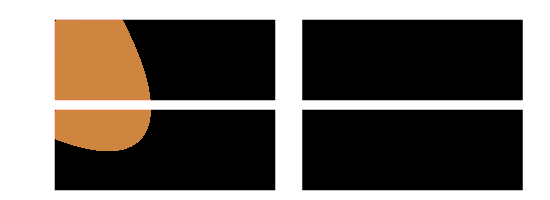<?xml version="1.0" standalone="no"?>
<!DOCTYPE svg PUBLIC "-//W3C//DTD SVG 1.1//EN" "http://www.w3.org/Graphics/SVG/1.1/DTD/svg11.dtd">

<svg viewBox = "0 0 1100 400" version = "1.1">
<desc>
Filter example
</desc>
<filter id = "i1">
<feGaussianBlur in = "SourceAlpha" stdDeviation = "8" result = "blur1"/>
<feDiffuseLighting result = "diffOut" in = "SourceGraphic" diffuseConstant = "20" lighting-color = "white">
<feSpotLight x = "800" y = "800" z = "400" pointsAtX = "0" pointsAtY = "0" pointsAtZ = "0" limitingConeAngle = "9"/>
</feDiffuseLighting>
<feComposite in = "SourceGraphic" in2 = "diffOut" operator = "arithmetic" k1 = "1" k2 = "0" k3 = "0" k4 = "0"/>
</filter>
<g stroke = "tomato" fill = "peru" filter = "url(#i1)">
<rect x = "10%" y = "10%" width = "40%" height = "40%"/>
<rect x = "55%" y = "10%" width = "40%" height = "40%"/>
<rect x = "10%" y = "55%" width = "40%" height = "40%"/>
<rect x = "55%" y = "55%" width = "40%" height = "40%"/>
</g>
</svg>